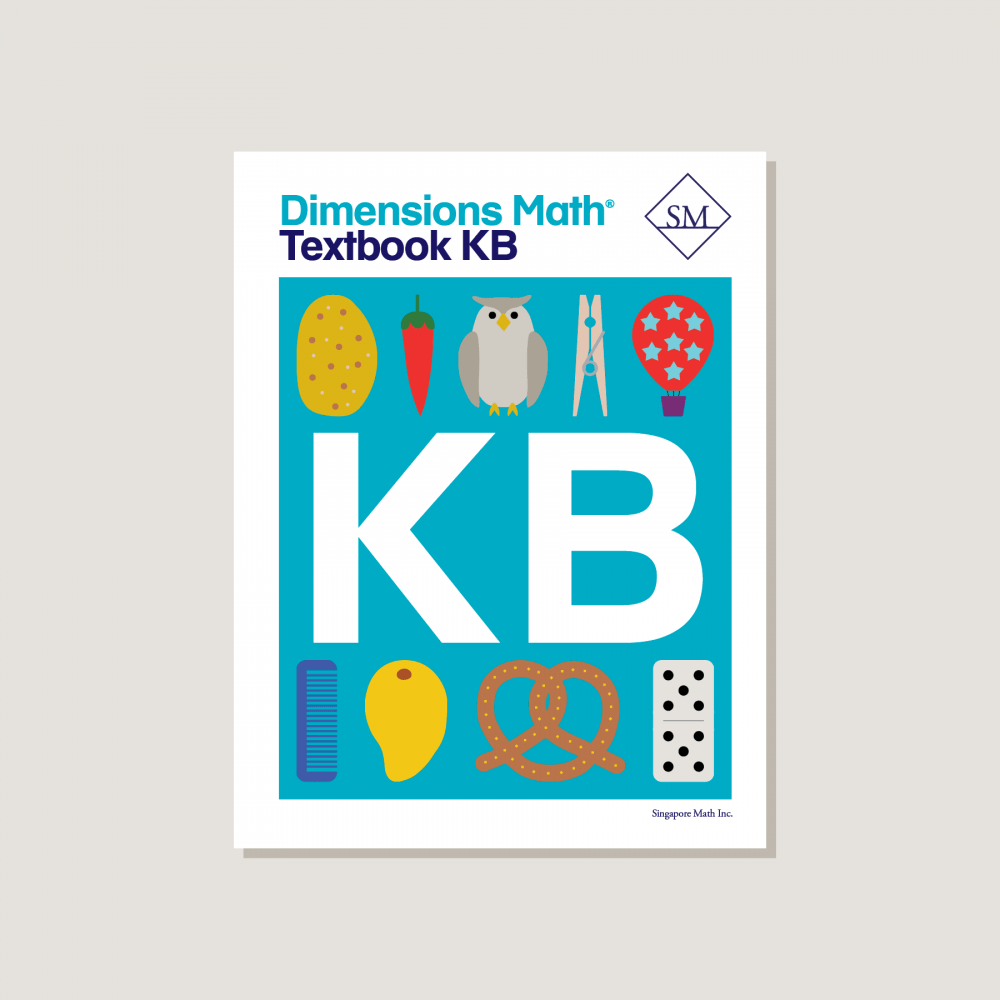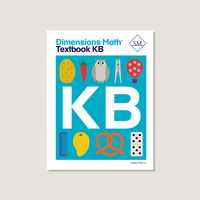Dimensions Math Textbook KB

\$12.80
Condition New

Textbooks develop concepts in increasing levels of abstraction. Each topic is thoughtfully introduced and extended through the use of exploration, play, and opportunities for mastery of skills. Features & Components:
• Chapter Opener: Each chapter begins with an engaging scenario that stimulates student curiosity in new concepts. This scenario also provides teachers an opportunity to review skills.
• Lesson: Engaging imagery draws students into the concept of each lesson.
• Exercise: A pencil icon at the end of the lesson links to additional practice problems in the corresponding workbook.
• Review: A review of chapter material provides ongoing practice of concepts and skills.
Note: Two textbooks (A and B) for each grade correspond to the two halves of the school year. Answer key not included. Soft cover.
SKU: DMTKB

ISBN: 9781947226036

Pagecount: 200

Dimensions: 8.5 x 11 x 0.75 in

Weight: 1.25 lbs

Binding: Standard

Color: Color

Cover: Soft

Perforated: No

Sample Pages

Chapter 7: Numbers to 20
Lesson 1: Ten and Some More
Lesson 2: Count Ten and Some More
Lesson 3: Two Ways to Count
Lesson 4: Numbers 16 to 20
Lesson 5: Number Words 0 to 10
Lesson 6: Number Words 11 to 15
Lesson 7: Number Words 16 to 20
Lesson 8: Number Order
Lesson 9: 1 More Than or Less Than
Lesson 10: Practice — Part 1
Lesson 11: Practice — Part 2

Chapter 8: Number Bonds
Lesson 1: Putting Numbers Together — Part 1
Lesson 2: Putting Numbers Together — Part 2
Lesson 3: Parts Making a Whole
Lesson 4: Look for a Part
Lesson 5: Number Bonds for 2, 3, and 4
Lesson 6: Number Bonds for 5
Lesson 7: Number Bonds for 6
Lesson 8: Number Bonds for 7
Lesson 9: Number Bonds for 8
Lesson 10: Number Bonds for 9
Lesson 11: Number Bonds for 10
Lesson 12: Practice — Part 1
Lesson 13: Practice — Part 2
Lesson 14: Practice — Part 3

Lesson 1: Introduction to Addition — Part 1
Lesson 2: Introduction to Addition — Part 2
Lesson 3: Introduction to Addition — Part 3
Lesson 5: Count On — Part 1
Lesson 6: Count On — Part 2
Lesson 7: Add Up to 3 and 4
Lesson 8: Add Up to 5 and 6
Lesson 9: Add Up to 7 and 8
Lesson 10: Add Up to 9 and 10
Lesson 12: Practice

Chapter 10: Subtraction
Lesson 1: Take Away to Subtract — Part 1
Lesson 2: Take Away to Subtract — Part 2
Lesson 3: Take Away to Subtract — Part 3
Lesson 4: Take Apart to Subtract — Part 1
Lesson 5: Take Apart to Subtract — Part 2
Lesson 6: Count Back
Lesson 7: Subtract Within 5
Lesson 8: Subtract Within 10 — Part 1
Lesson 9: Subtract Within 10 — Part 2
Lesson 10: Practice

Lesson 2: Practice Addition and Subtraction
Lesson 3: Part-Whole Addition and Subtraction
Lesson 4: Add to or Take Away
Lesson 5: Put Together or Take Apart
Lesson 6: Practice

Chapter 12: Numbers to 100
Lesson 1: Count by Tens — Part 1
Lesson 2: Count by Tens — Part 2
Lesson 3: Numbers to 30
Lesson 4: Numbers to 40
Lesson 5: Numbers to 50
Lesson 6: Numbers to 80
Lesson 7: Numbers to 100 — Part 1
Lesson 8: Numbers to 100 — Part 2
Lesson 9: Count by Fives — Part 1
Lesson 10: Count by Fives — Part 2
Lesson 11: Practice

Chapter 13: Time
Lesson 1: Day and Night
Lesson 2: Learning About the Clock
Lesson 3: Telling Time to the Hour — Part 1
Lesson 4: Telling Time to the Hour — Part 2
Lesson 5: Practice

Chapter 14: Money
Lesson 1: Coins
Lesson 2: Pennies
Lesson 3: Nickels
Lesson 4: Dimes
Lesson 5: Quarters
Lesson 6: Practice

A & B Books: Our programs divide the school year into two semesters. “A” level books are for the first half of the school year. “B” level books are for the second half of the school year. You need both “A” and “B” material for a complete school year.

Required Components: Textbooks, Workbooks, and Guides (either Home Instructor’s Guides or Teacher’s Guides) are all necessary components. These three elements each serve a unique function and work together to build math mastery.

Printouts: Dimensions Math PK-5 Resources

Recommended Manipulatives: Dimensions Math Grades PK-5 Recommended Manipulatives

Answer Key: Located in corresponding Home Instructor’s Guide/Teacher’s Guide

Dimensions Math At Home™ Videos

Invite a professional Singapore math teacher into your home classroom. This subscription of pre-recorded lessons covers all Textbook and Workbook material for an entire school year and can be done at your own pace.

Manipulatives

Manipulatives help students visualize and represent math concepts. Stock up on suggested items to deepen engagement during math activities and lessons.

Supplementary Math

Supplementary math helps students of all abilities: struggling, advanced, or on level. Find just the right addition to round out your core program.